# Tutorial

Below is a quick tutorial to help get you started. Note that we assume you have Julia installed and configured already.

## Getting Makie

Enter the package manager by typing ] into the Repl. You should see pkg>.

add Makie

Run the following commands in the package manager:

add Makie#master AbstractPlotting#master GLMakie#master
test Makie

The first use of Makie might take a little bit of time, due to precompilation.

## Set the Scene

The Scene object holds everything in a plot, and you can initialize it like so:

scene = Scene()

Note that before you put anything in the scene, it will be blank!

## Getting help

The user-facing functions of Makie are pretty well documented, so you can usually use the help mode in the REPL, or your editor of choice. If you countinue to have issues, see Getting Help.

## Basic plotting

Below are some examples of basic plots to help you get oriented.

You can put your mouse in the plot window and scroll to zoom. Right click and drag lets you pan around the scene, and left click and drag lets you do selection zoom (in 2D plots), or orbit around the scene (in 3D plots).

Many of these examples also work in 3D.

It is worth noting initally that if you run a Makie.jl example and nothing shows up, you likely need to do display(scene) to render the example on screen.

### Scatter plot

using Makie

x = rand(10)
y = rand(10)
colors = rand(10)
scene = scatter(x, y, color = colors)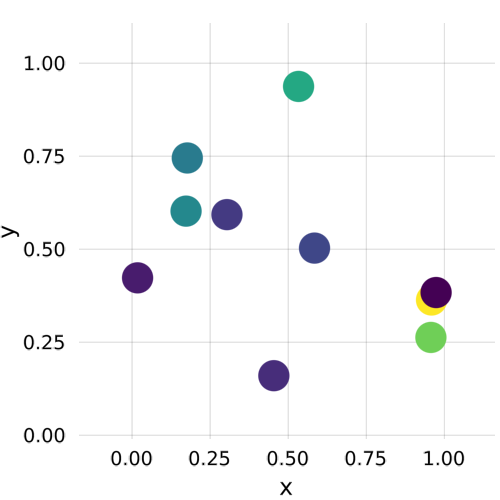using Makie

x = 1:10
y = 1:10
sizevec = [s for s = 1:length(x)] ./ 10
scene = scatter(x, y, markersize = sizevec)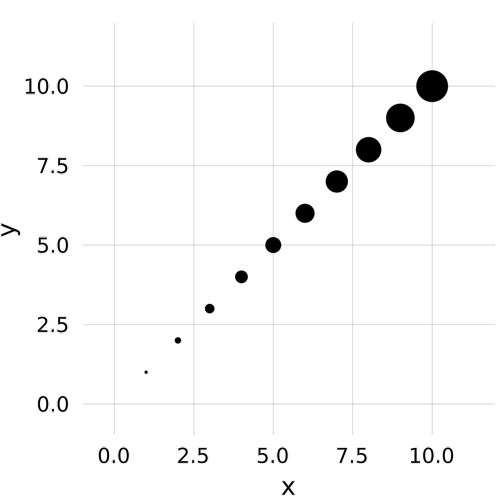### Line plot

using Makie

x = range(0, stop = 2pi, length = 40)
f(x) = sin.(x)
y = f(x)
scene = lines(x, y, color = :blue)using Makie

scene = lines(rand(10))

sc_t = title(scene, "Random lines")

sc_t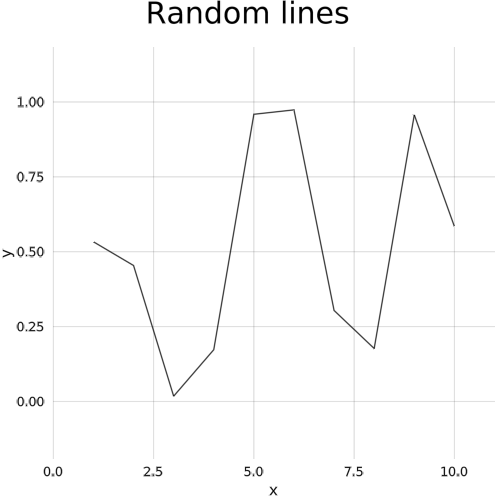using Makie

x = range(0, stop = 2pi, length = 80)
f1(x) = sin.(x)
f2(x) = exp.(-x) .* cos.(2pi*x)
y1 = f1(x)
y2 = f2(x)

scene = lines(x, y1, color = :blue)
scatter!(scene, x, y1, color = :red, markersize = 0.1)

lines!(scene, x, y2, color = :black)
scatter!(scene, x, y2, color = :green, marker = :utriangle, markersize = 0.1)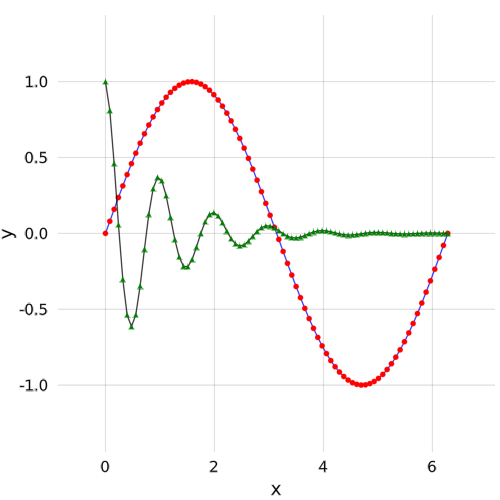### Removing from a scene

using Makie

x = range(0, stop = 2pi, length = 80)
f1(x) = sin.(x)
f2(x) = exp.(-x) .* cos.(2pi*x)
y1 = f1(x)
y2 = f2(x)

scene = lines(x, y1, color = :blue)
scatter!(scene, x, y1, color = :red, markersize = 0.1)

lines!(scene, x, y2, color = :black)
scatter!(scene, x, y2, color = :green, marker = :utriangle, markersize = 0.1)
# initialize the stepper and give it an output destination
st = Stepper(scene, "tutorial_removing_from_a_scene")
step!(st)

pop!(scene.plots)
step!(st)

pop!(scene.plots)
step!(st)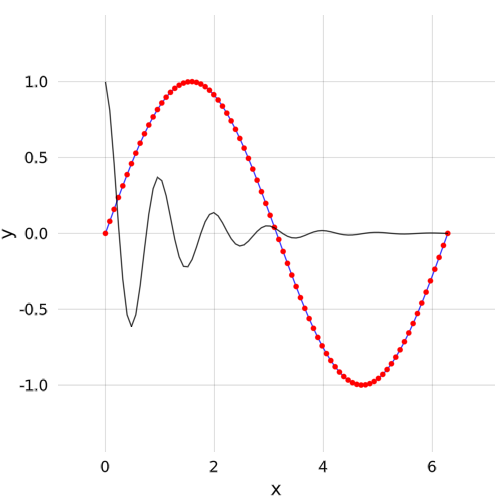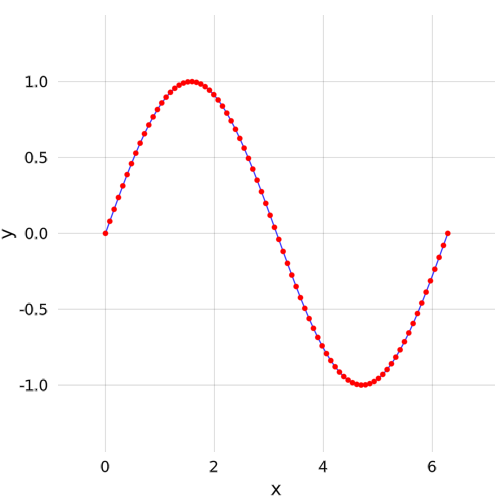using Makie

x = range(0, stop = 10, length = 40)
y = x
#= specify the scene limits, note that the arguments for FRect are
x_min, y_min, x_dist, y_dist,
therefore, the maximum x and y limits are then x_min + x_dist and y_min + y_dist
=#
limits = FRect(-5, -10, 20, 30)

scene = lines(x, y, color = :blue, limits = limits)You can also use the convenience functions xlims!, ylims! and zlims!.

### Basic theming

using Makie

x = range(0, stop = 2pi, length = 40)
f(x) = cos.(x)
y = f(x)
scene = lines(x, y, color = :blue)

axis = scene[Axis] # get the axis object from the scene
axis.grid.linecolor = ((:red, 0.5), (:blue, 0.5))
axis.names.textcolor = ((:red, 1.0), (:blue, 1.0))
axis.names.axisnames = ("x", "y = cos(x)")
scene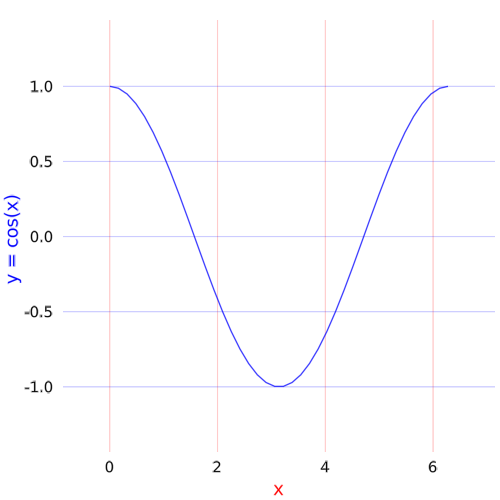### Statistical plotting

Makie has a lot of support for statistical plots through StatsMakie.jl. See the StatsMakie Tutorial section for more information on this.

## Controlling display programmatically

Scenes will only display by default in global scope. To make a Scene display when it's defined in a local scope, like a function or a module, you can call display(scene), which will automatically display it in the best available display. You can force display to the backend's preferred window by calling display(AbstractPlotting.PlotDisplay(), scene).

## Saving plots

See the Output section.

## Animations

See the Animation section, as well as the Interaction section.

## More examples

See the Example Gallery.